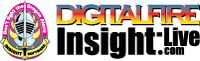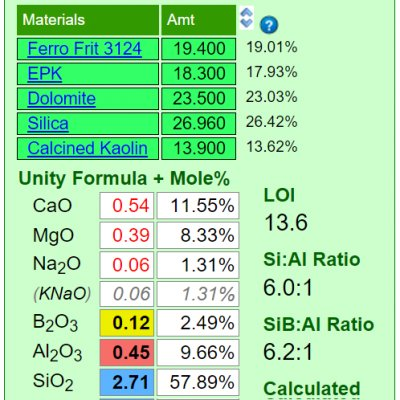•The secret to cool bodies and glazes is a lot of testing.
•The secret to know what to test is material and chemistry knowledge.
•The secret to learning from testing is documentation.
•The place to test, do the chemistry and document is an account at https://insight-live.com
•The place to get the knowledge is https://digitalfire.com

Sign-up at https://insight-live.com today.

Unity Formula

A "unity formula" is just a formula that has been retotaled so that the RO group of oxides totals one. This is also called a Seger formula and this standard provides one basis for comparing glazes. The three column format of expressing a formula was first used by Hermann Seger. Here is how we would recalculate a raw formula to a flux unity:

Raw              Unity
Oxides Formula Formula
-----------------------------------
K2O 0.6 / 2.2 = 0.27
CaO 1.3 / 2.2 = 0.59
MgO 0.2 / 2.2 = 0.09
ZnO 0.1 / 2.2 = 0.05
----- ----
Flux Total 2.2 1.0
Al2O3 0.9 / 2.2 = 0.41
SiO2 9.0 / 2.2 = 4.09
-----------------------------------

The Seger method of rationalizing the chemistry of glazes does not work as well at lower temperatures because some oxides that are powerful fluxes at high temperatures are refractory in low fire. Oxides have a much more individual presence (at each temperature range) than the Seger method tends to recognize. Also, their contributions to particular properties often are not linear according to concentration. For example, boron is both a glass and a flux and the logic for its employment at various temperature ranges differs. It does not 'plug into' a Seger formula well. Many people prefer to use Mole% when rationalizing how a formula relates to the fired product. However, all of this being said, there is great value in a method of presentation that clearly shows the relationship between Al2O3, SiO2 and everything else.

Some argue that the unity formula method of presenting glazes is not precise enough for glaze chemistry calculations. However, the greatest source of imprecision by far is the accuracy of the analyses of the materials being used. Any method that calculates an exact match for an existing chemistry will also produce an exact match in unity formulas. Unity formulas work better in targeting when doing batch to chemistry calculations because additions or changes in percentage of a material do not always change every number in the formula. However it must be remembered that glaze chemistry is not about precise mathematics, it is comparative and best viewed in context and as a tool to help when comparing one thing with another.

Digitalfire desktop Insight required that users specify whether to unify recipes to RO or R2O3. However Insight-live automatically sets this (the group having the greater total wins the unity setting). Users can click the 'Auto Unity' heading and it will cycle between various settings, eventually coming back to auto-unity. Recipes remember their settings.

A Limitation of the Seger Unity FormulaMineral sources of oxides impose their own melting patterns and when one is substituted for another to supply an oxide a different system with its own relative chemistry is entered. An extreme example of this would be to source Al2O3 to a glaze using calcined alumina instead of kaolin. Although the formula may be exactly the same, the fired result would be completely different because very little of the alumina would dissolve into the glaze melt. At the opposite extreme, a different frit could be used to supply a set of oxides (while maintaining the overall chemistry of the glaze) and the fired result would be much more chemically predictable. Why? Because the readily and release their oxides the the melt.

• (Articles) Glaze Chemistry Basics - Formula, Analysis, Mole%, Unity, LOI

Part of changing your viewpoint of glazes, from a collection of materials to a collection of oxides, is learning what a formula and analysis are, how conversion between the two is done and how unity and LOI impact this.

• (Articles) How INSIGHT Deals With Unity, LOI and Formula Weight

INSIGHT enables you to enter material analyses as recipes as a first step to inserting them into the materials database. Imposing an LOI and understanding how to set unity and its connection for formula weight are important concepts.

• (Glossary) Glaze Chemistry

Glaze chemistry is learning what each oxide does in a fired glaze and the relative advantages and disadvantages of each material supplying it. The chemistry of a glaze is expressed in a manner similar to its recipe, except that the items are oxides and the amounts can be by weight (an analysis) or n...

• (Glossary) Mole%

Mole% is a way of expressing the oxide formula of a fired glaze or glass (technicians can extrapolate fired properties like melting temperature, thermal expansion, hardness, resistance to leaching, etc. by examining the chemistry of a glaze). Mole% is preferred over the Seger unity formula by many t...

• (Glossary) Analysis

Conceptually we consider fired glazes as being composed of 'oxides'. Materials supply those oxides to the melt. The ten major oxides likely make up 99% of all base glazes (and materials we use). The oxide formula of a glaze "explains" many details about the way the glaze fires. Thus we can view m...

• (Glossary) LOI

Simplistically, LOI is the percentage of weight a material loses on firing. Assuming firing to a typical stoneware temperature of 1200C, the amount of weight loss can be surprising. Kaolins, for example, lose around 12% (mainly crystal-bound water). Ball clays lose about half of that (a combination ...

• (Glossary) Formula

Conceptually we consider fired ceramic glazes as being composed of 'oxides' (materials contribute these). The ten major oxides likely make up 99% of all base glazes (and materials we use). The oxide formula of a glaze "explains" many details about the way the glaze fires (provided all the materials ...

• (Videos) Lesson 5A - Glaze Formula to Batch Calculations

Shows you how to use a non-unity calculation and the supply button to convert a formula into a batch recipe. You will find out how to match a target formula exactly using theoretical materials and how...